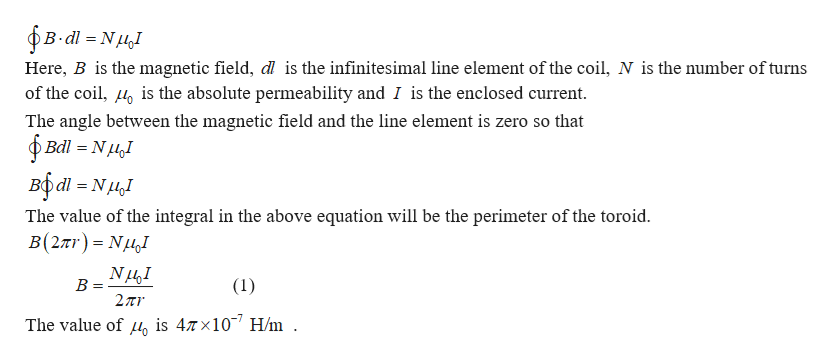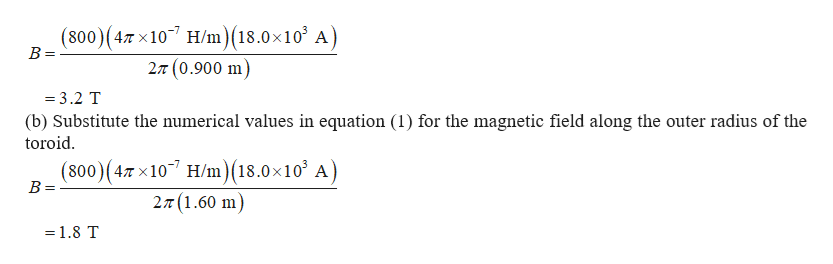# The magnetic coils of a tokamak fusion reactor are in theshape of a toroid having an inner radius of 0.900 m and an outer radiusof 1.60 m. The toroid has 800 turns of large-diameter wire, each of whichcarries a current of 18.0 kA. Find the magnitude of the magnetic fieldinside the toroid along (a) the inner radius and (b) the outer radius.

Question
22 views

The magnetic coils of a tokamak fusion reactor are in the
shape of a toroid having an inner radius of 0.900 m and an outer radius
of 1.60 m. The toroid has 800 turns of large-diameter wire, each of which
carries a current of 18.0 kA. Find the magnitude of the magnetic field
inside the toroid along (a) the inner radius and (b) the outer radius.

check_circle

Step 1

Ampere’s circuital law can be used to find the magnitude of the magnetic field. The law is given byhelp_outlineImage TranscriptioncloseB-dl N Here, B is the magnetic field, dl is the infinitesimal line element of the coil, N is the mumber of turns of the coil, is the absolute permeability and I is the enclosed current The angle between the magnetic field and the line element is zero so that Bdl NI вбаі - Nн,1 The value of the integral in the above equation will be the perimeter of the toroid в(2лr) %3 NuJ NI В - 2π (1) The value of is 47x10 H/m fullscreen
Step 2

(a)Substitute the numerical values in equation (1) for the ...help_outlineImage Transcriptionclose(800) (47 x 107 H/m)(18.0x103 A) B = 27 (0.900 m =3.2 T (b) Substitute the numerical values in equation (1) for the magnetic field along the outer radius of the toroid (800) (4,7x107 H/m)(18.0x10 A) 2π(1.60 m) B = =1.8 T fullscreen

### Want to see the full answer?

See Solution

#### Want to see this answer and more?

Solutions are written by subject experts who are available 24/7. Questions are typically answered within 1 hour.*

See Solution
*Response times may vary by subject and question.
Tagged in

### Magnetic Effects of Current